# Excel formulas for conditional formatting based on another cell value

In this tutorial, we will continue exploring the fascinating world of Excel Conditional Formatting. If you do not feel very comfortable in this area, you may want to look through the previous article first to revive the basics - How to use conditional formatting in Excel.

Today are going to dwell on how to use Excel formulas to format individual cells and entire rows based on the values you specify or based on another cell's value. This is often considered advanced aerobatics of Excel conditional formatting and once mastered, it will help you push the formats in your spreadsheets far beyond their common uses.

## Excel formulas for conditional formatting based on cell value

Excel's pre-defined conditional formatting rules are mainly purposed to format cells based on their own values or the values you specify. I am talking about Data Bars, Color Scales, Icon Sets and other rules available to you on the Conditional Formatting button click.

If you want to apply conditional formatting based on another cell or format the entire row based on a single cell's value, then you will need to use Excel formulas. So, let's see how you can make a rule using a formula and after that I will provide a number of formula examples for different tasks.

### How to create a conditional formatting rule using a formula

To set up a conditional formatting rule based on a formula in Excel 2019, Excel 2016, Excel 2013 and Excel 2010, carry out these steps:

1. Select the cells you want to format. You can select one column, several columns or the entire table if you want to apply your conditional format to rows.

Tip. If you plan to add more data in the future and you want the conditional formatting rule to get applied to new entries automatically, you can either:

• Convert a range of cells to a table (Insert tab > Table). In this case, the conditional formatting will be automatically applied to all new rows.
• Select some empty rows below your data, say 100 blank rows.
2. On the Home tab, in the Styles group, click Conditional formatting > New Rule…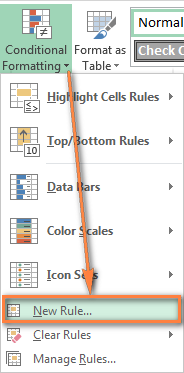3. In the New Formatting Rule window, select Use a formula to determine which cells to format.
4. Enter the formula in the corresponding box.
5. Click the Format… button to choose your custom format.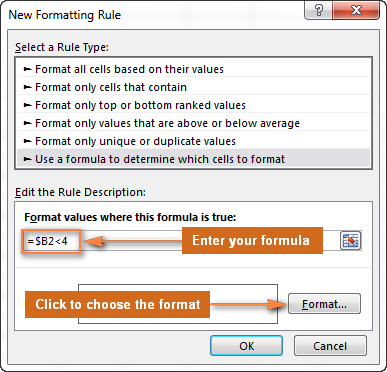6. Switch between the Font, Border and Fill tabs and play with different options such as font style, pattern color and fill effects to set up the format that works best for you. If the standard palette does not suffice, click More colors… and choose any RGB or HSL color to your liking. When done, click the OK button.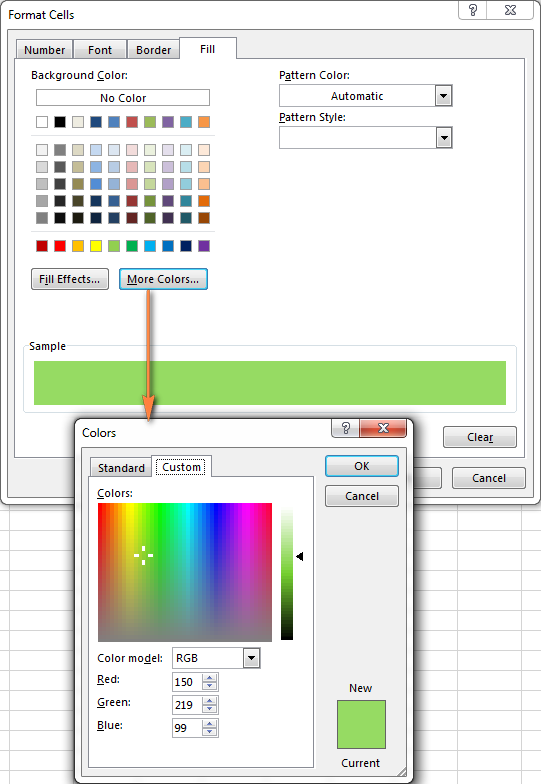7. Make sure the Preview section displays the format you want and if it does, click the OK button to save the rule. If you are not quite happy with the format preview, click the Format… button again and make the edits.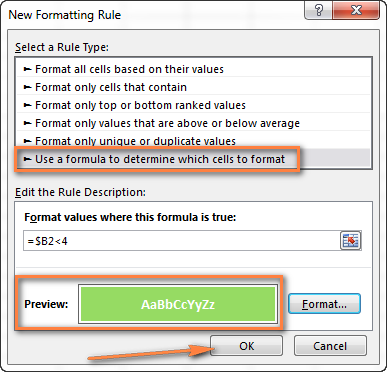Tip. Whenever you need to edit a conditional formatting formula, press F2 and then move to the needed place within the formula using the arrow keys. If you try arrowing without pressing F2, a range will be inserted into the formula rather than just moving the insertion pointer. To add a certain cell reference to the formula, press F2 a second time and then click that cell.

## Excel conditional formatting formula examples

Now that you know how to create and apply Excel conditional formatting based on another cell, let's move on and see how to use various Excel formulas in practice:

### Formulas to compare values (numbers and text)

As you know Microsoft Excel provides a handful of ready-to-use rules to format cells with values greater than, less than or equal to the value you specify (Conditional Formatting >Highlight Cells Rules). However, these rules do not work if you want to conditionally format certain columns or entire rows based on a cell's value in another column. In this case, you use analogous formulas:

 Condition Formula example Equal to `=\$B2=10` Not equal to `=\$B2<>10` Greater than `=\$B2>10` Greater than or equal to `=\$B2>=10` Less than `=\$B2<10` Less than or equal to `=\$B2<=10` Between `=AND(\$B2>5, \$B2<10)`

The screenshot below shows an example of the Greater than formula that highlights product names in column A if the number of items in stock (column C) is greater than 0. Please pay attention that the formula applies to column A only (\$A\$2:\$A\$8). But if you select the whole table (in our case, \$A\$2:\$E\$8), this will highlight entire rows based on the value in column C.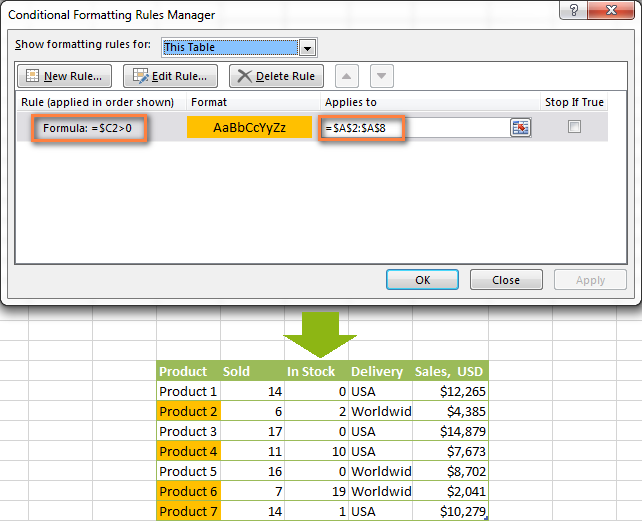In a similar fashion, you can create a conditional formatting rule to compare values of two cells. For example:

`=\$A2<\$B2` - format cells or rows if a value in column A is less than the corresponding value in column B.

`=\$A2=\$B2` - format cells or rows if values in columns A and B are the same.

`=\$A2<>\$B2` - format cells or rows if a value in column A is not the same as in column B.

As you can see in the screenshot below, these formulas work for text values as well as for numbers.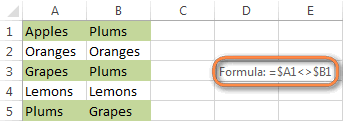### AND and OR formulas

If you want to format your Excel table based on 2 or more conditions, then use either =AND or =OR function:

 Condition Formula Description If both conditions are met `=AND(\$B2<\$C2, \$C2<\$D2)` Formats cells if the value in column B is less than in column C, and if the value in column C is less than in column D. If one of the conditions is met `=OR(\$B2<\$C2, \$C2<\$D2)` Formats cells if the value in column B is less than in column C, or if the value in column C is less than in column D.

In the screenshot below, we use the formula `=AND(\$C2>0, \$D2="Worldwide")` to change the background color of rows if the number of items in stock (Column C) is greater than 0 and if the product ships worldwide (Column D). Please pay attention that the formula works with text values as well as with numbers.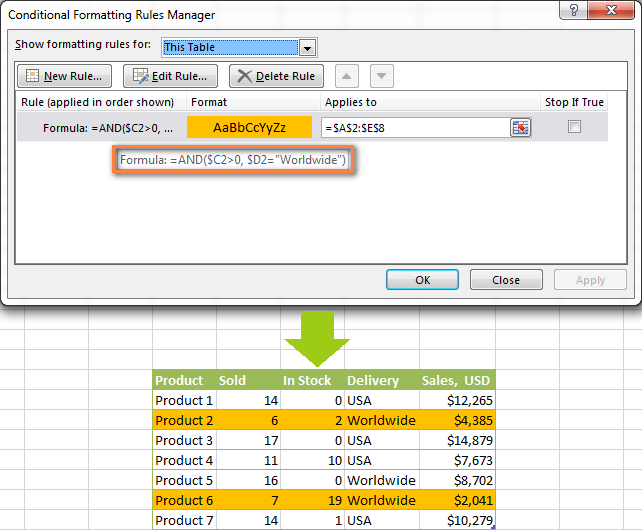Naturally, you can use two, three or more conditions in your =AND and =OR formulas.

These are the basic conditional formatting formulas you use in Excel. Now let's consider a bit more complex but far more interesting examples.

### Conditional formatting for empty and non-empty cells

I think everyone knows how to format empty and not empty cells in Excel - you simply create a new rule of the "Format only cells that contain" type and choose either Blanks or No Blanks.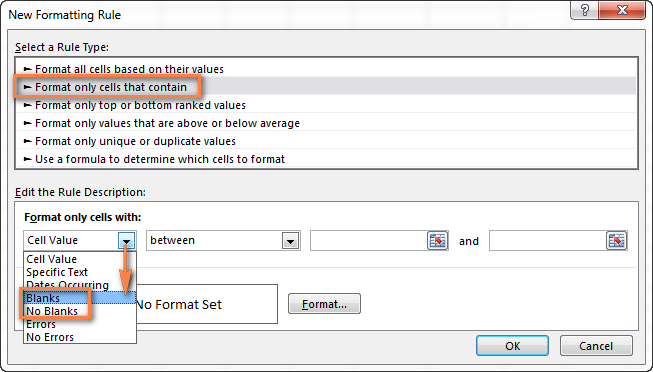But what if you want to format cells in a certain column if a corresponding cell in another column is empty or not empty? In this case, you will need to utilize Excel formulas again:

Formula for blanks: `=\$B2=""` - format selected cells / rows if a corresponding cell in Column B is blank.

Formula for non-blanks: `=\$B2<>""` - format selected cells / rows if a corresponding cell in Column B is not blank.

Note. The formulas above will work for cells that are "visually" empty or not empty. If you use some Excel function that returns an empty string, e.g. `=if(false,"OK", "")`, and you don't want such cells to be treated as blanks, use the following formulas instead `=isblank(A1)=true` or `=isblank(A1)=false` to format blank and non-blank cells, respectively.

And here is an example of how you can use the above formulas in practice. Suppose, you have a column (B) which is "Date of Sale" and another column (C) "Delivery". These 2 columns have a value only if a sale has been made and the item delivered. So, you want the entire row to turn orange when you've made a sale; and when an item is delivered, a corresponding row should turn green. To achieve this, you need to create 2 conditional formatting rules with the following formulas:

• Orange rows (a cell in column B is not empty): `=\$B2<>""`
• Green rows (cells in column B and column C are not empty): `=AND(\$B2<>"", \$C2<>"")`

One more thing for you to do is to move the second rule to the top and select the Stop if true check box next to this rule: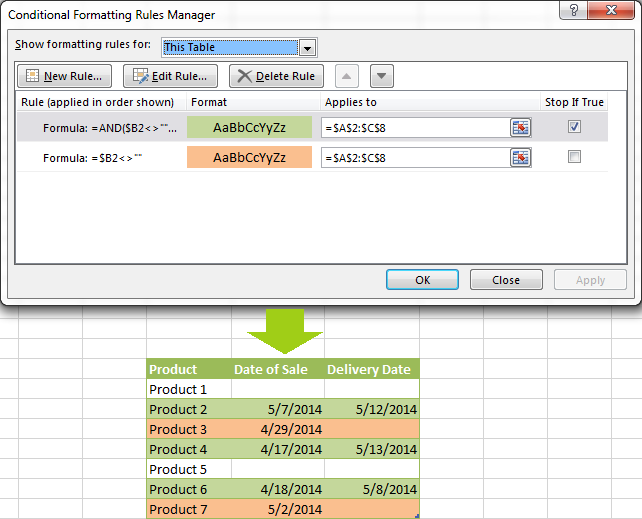In this particular case, the "Stop if true" option is actually superfluous, and the rule will work with or without it. You may want to check this box just as an extra precaution, in case you add a few other rules in the future that may conflict with any of the existing ones.

### Excel formulas to work with text values

If you want to format a certain column(s) when another cell in the same row contains a certain word, you can use a formula discussed in one of the previous examples (like =\$D2="Worldwide"). However, this will only work for exact match.

For partial match, you will need to use either SEARCH (case insensitive) or FIND (case sensitive).

For example, to format selected cells or rows if a corresponding cell in column D contains the word "Worldwide", use the below formula. This formula will find all such cells, regardless of where the specified text is located in a cell, including "Ships Worldwide", "Worldwide, except for…", etc:

`=SEARCH("Worldwide", \$D2)>0`

If you'd like to shade selected cells or rows if the cell's content starts with the search text, use this one:

`=SEARCH("Worldwide", \$D2)>1`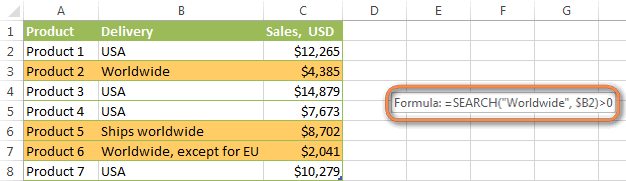### Excel formulas to highlight duplicates

If your task is to conditionally format cells with duplicate values, you can go with the pre-defined rule available under Conditional formatting > Highlight Cells Rules > Duplicate Values… The following article provides a detailed guidance on how to use this feature: How to automatically highlight duplicates in Excel.

However, in some cases the data looks better if you color selected columns or entire rows when a duplicate values occurs in another column. In this case, you will need to employ an Excel conditional formatting formula again, and this time we will be using the COUNTIF formula. As you know, this Excel function counts the number of cells within a specified range that meet a single criterion.

#### Highlight duplicates including 1st occurrences

`=COUNTIF(\$A\$2:\$A\$10,\$A2)>1` - this formula finds duplicate values in the specified range in Column A (A2:A10 in our case), including first occurrences.

If you choose to apply the rule to the entire table, the whole rows will get formatted, as you see in the screenshot below. I've decided to change a font color in this rule, just for a change : )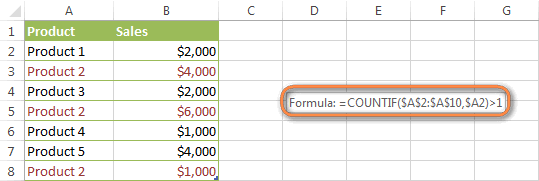#### Highlight duplicates without 1st occurrences

To ignore the first occurrence and highlight only subsequent duplicate values, use this formula: `=COUNTIF(\$A\$2:\$A2,\$A2)>1`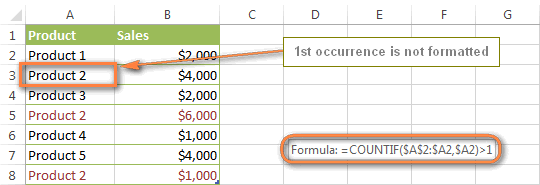#### Highlight consecutive duplicates in Excel

If you'd rather highlight only duplicates on consecutive rows, you can do this in the following way. This method works for any data types: numbers, text values and dates.

• Select the column where you want to highlight duplicates, without the column header.
• Create a conditional formatting rule(s) using these simple formulas:
Rule 1 (blue): `=\$A1=\$A2` - highlights the 2nd occurrence and all subsequent occurrences, if any.
Rule 2 (green): `=\$A2=\$A3` - highlights the 1st occurrence.

In the above formulas, A is the column you want to check for dupes, \$A1 is the column header, \$A2 is the first cell with data.

Important! For the formulas to work correctly, it is essential that Rule 1, which highlights the 2nd and all subsequent duplicate occurrences, should be the first rule in the list, especially if you are using two different colors.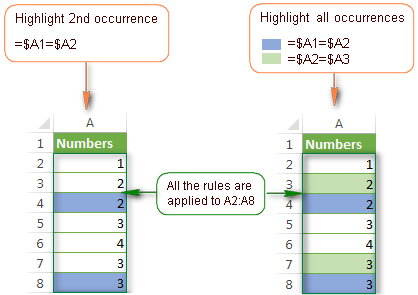#### Highlight duplicate rows

If you want apply the conditional format when duplicate values occur in two or more columns, you will need to add an extra column to your table in which you concatenate the values from the key columns using a simple formula like this one `=A2&B2`. After that you apply a rule using either variation of the COUNTIF formula for duplicates (with or without 1st occurrences). Naturally, you can hide an additional column after creating the rule.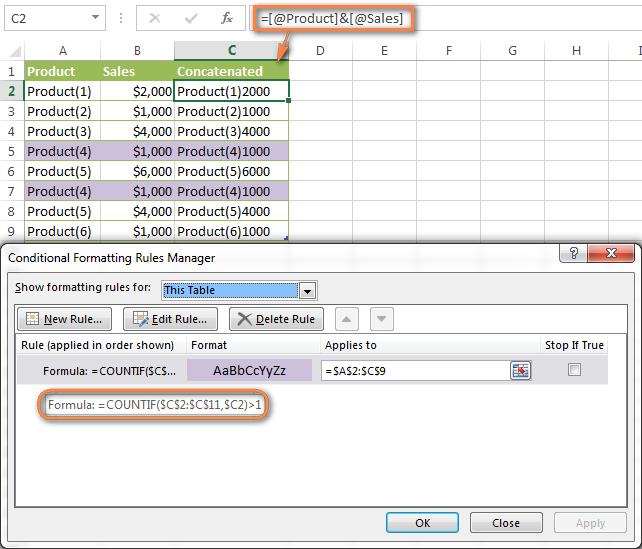Alternatively, you can use the COUNTIFS function that supports multiple criteria in a single formula. In this case, you won't need a helper column.

In this example, to highlight duplicate rows with 1st occurrences, create a rule with the following formula:
`=COUNTIFS(\$A\$2:\$A\$11, \$A2, \$B\$2:\$B\$11, \$B2)>1`

To highlight duplicate rows without 1st occurrences, use this formula:
`=COUNTIFS(\$A\$2:\$A2, \$A2, \$B\$2:\$B2, \$B2)>1`

#### Compare 2 columns for duplicates

One of the most frequent tasks in Excel is to check 2 columns for duplicate values - i.e. find and highlight values that exist in both columns. To do this, you will need to create an Excel conditional formatting rule for each column with a combination of `=ISERROR()` and `=MATCH()` functions:

For Column A: `=ISERROR(MATCH(A1,\$B\$1:\$B\$10000,0))=FALSE`

For Column B: `=ISERROR(MATCH(B1,\$A\$1:\$A\$10000,0))=FALSE`

Note. For such conditional formulas to work correctly, it's very important that you apply the rules to the entire columns, e.g. `=\$A:\$A` and `=\$B:\$B`.

You can see an example of practical usage in the following screenshot that highlights duplicates in Columns E and F.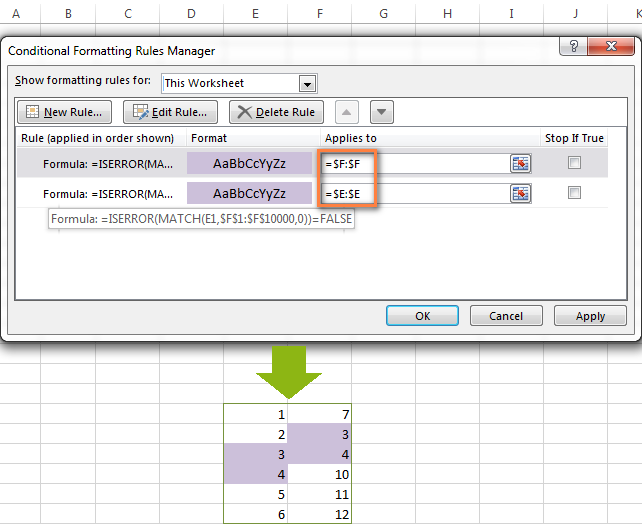As you can see, Excel conditional formatting formulas cope with dupes pretty well. However, for more complex cases, I would recommend using the Duplicate Remover add-in that is especially designed to find, highlight and remove duplicates in Excel, in one sheet or between two spreadsheets.

### Formulas to highlight values above or below average

When you work with several sets of numeric data, the AVERAGE() function may come in handy to format cells whose values are below or above the average in a column.

For example, you can use the formula `=\$E2<AVERAGE(\$E\$2:\$E\$8)` to conditionally format the rows where the sale numbers are below the average, as shown in the screenshot below. If you are looking for the opposite, i.e. to shade the products performing above the average, replace "<" with ">" in the formula: `=\$E2>AVERAGE(\$E\$2:\$E\$8)`.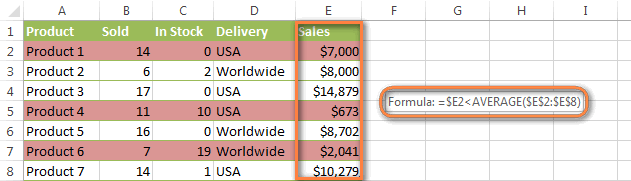### How to highlight the nearest value in Excel

If I have a set of numbers, is there a way I can use Excel conditional formatting to highlight the number in that set that is closest to zero? This is what one of our blog readers, Jessica, wanted to know. The question is very clear and straightforward, but the answer is a bit too long for the comments sections, that's why you see a solution here :)

#### Example 1. Find the nearest value, including exact match

In our example, we'll find and highlight the number that is closest to zero. If the data set contains one or more zeroes, all of them will be highlighted. If there is no 0, then the value closest to it, either positive or negative, will be highlighted.

First off, you need to enter the following formula to any empty cell in your worksheet, you will be able to hide that cell later, if needed. The formula finds the number in a given range that is closest to the number you specify and returns the absolute value of that number (absolute value is the number without its sign):

`=MIN(ABS(B2:D13-(0)))`

In the above formula, B2:D13 is your range of cells and 0 is the number for which you want to find the closest match. For example, if you are looking for a value closest to 5, the formula will change to: `=MIN(ABS(B2:D13-(5)))`

Note. This is an array formula, so you need to press Ctrl + Shift + Enter instead of a simple Enter stroke to complete it.

And now, you create a conditional formatting rule with the following formula, where B3 is the top-right cell in your range and \$C\$2 in the cell with the above array formula:

`=OR(B3=0-\$C\$2,B3=0+\$C\$2)`

Please pay attention to the use of absolute references in the address of the cell containing the array formula (\$C\$2), because this cell is constant. Also, you need to replace 0 with the number for which you want to highlight the closest match. For example, if we wanted to highlight the value nearest to 5, the formula would change to: `=OR(B3=5-\$C\$2,B3=5+\$C\$2)`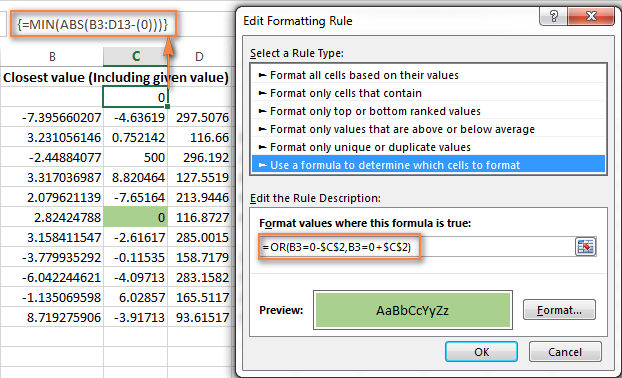#### Example 2. Highlight a value closest to the given value, but NOT exact match

In case you do not want to highlight the exact match, you need a different array formula that will find the closest value but ignore the exact match.

For example, the following array formula finds the value closest to 0 in the specified range, but ignores zeroes, if any:

`=MIN(ABS(B3:C13-(0))+(10^0*(B3:C13=0)))`

Please remember to press Ctrl + Shift + Enter after you finished typing your array formula.

The conditional formatting formula is the same as in the above example:

`=OR(B3=0-\$C\$2,B3=0+\$C\$2)`

However, since our array formula in cell C2 ignores the exact match, the conditional formatting rule ignores zeroes too and highlights the value 0.003 that is the closest match.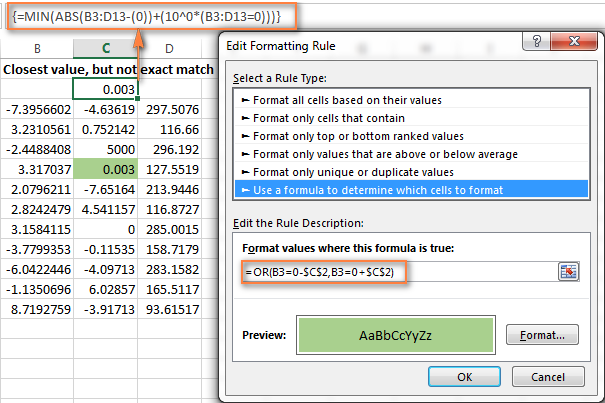If you want to find the value nearest to some other number in your Excel sheet, just replace "0" with the number you want both in the array and conditional formatting formulas.

I hope the conditional formatting formulas you have learned in this tutorial will help you make sense of whatever project you are working on. If you need more examples, please check out the following articles:

## Why isn't my Excel conditional formatting working correctly?

If your conditional formatting rule is not working as expected, though the formula is apparently correct, do not get upset! Most likely it is not because of some weird bug in Excel conditional formatting, rather due to a tiny mistake, not evident at the first sight. Please try out 6 simple troubleshooting steps below and I'm sure you will get your formula to work:

1. Use absolute & relative cell addresses correctly. It's very difficult to deduce a general rule that will work in 100 per cent of cases. But most often you would use an absolute column (with \$) and relative row (without \$) in your cell references, e.g. `=\$A1>1`.

Please keep in mind that the formulas `=A1=1`, `=\$A\$1=1` and `=A\$1=1` will produce different results. If you are not sure which one is correct in your case, you can try all : ) For more information, please see Relative and absolute cell references in Excel conditional formatting.

2. Verify the applied range. Check whether your conditional formatting rule applies to the correct range of cells. A rule of thumb is this - select all the cells / rows you want to format but do not include column headers.
3. Write the formula for the top-left cell. In conditional formatting rules, cell references are relative to the top-left most cell in the applied range. So, always write your conditional formatting formula for the 1st row with data.

For example, if your data starts in row 2, you put `=A\$2=10` to highlight cells with values equal to 10 in all the rows. A common mistake is to always use a reference to the first row (e.g. `=A\$1=10`). Please remember, you reference row 1 in the formula only if your table does not have headers and your data really starts in row 1. The most obvious indication of this case is when the rule is working, but formats values not in the rows it should.

4. Check the rule you created. Double-check the rule in the Conditional Formatting Rules Manager. Sometimes, for no reason at all, Microsoft Excel distorts the rule you have just created. So, if the rule is not working, go to Conditional Formatting > Manage Rules and check both the formula and the range it applies to. If you have copied the formula from the web or some other external source, make sure the straight quotes are used.
5. Adjust cell references when copying the rule. If you copy Excel conditional formatting using Format Painter, don't forget to adjust all cell references in the formula.
6. Split complex formulas into simple elements. If you use a complex Excel formula that includes several different functions, split it into simple elements and verify each function individually.

And finally, if you've tried all the steps but your conditional formatting rule is still not working correctly, drop me a line in comments and we will try to fathom it out together :)

In my next article we are going to look into the capabilities of Excel conditional formatting for dates. See you next week and thanks for reading!